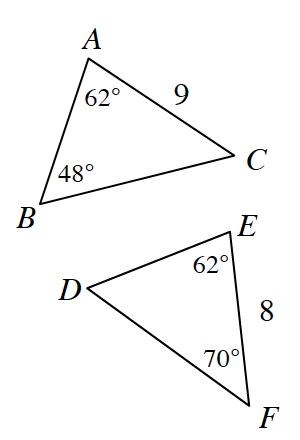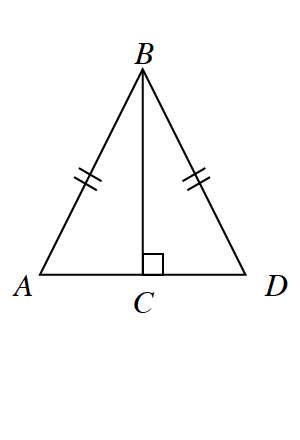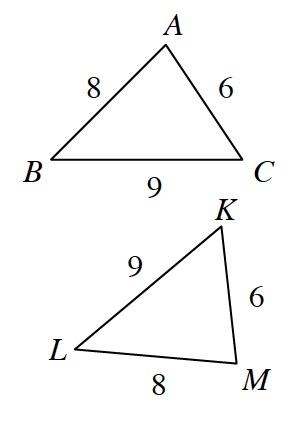### Home > GC > Chapter 9 > Lesson 9.2.1 > Problem9-61

9-61.

For each pair of triangles below, determine if the triangles are congruent. If they are congruent, state the congruence property that assures their congruence and write a congruence statement (such as $ΔABC ≅ Δ\text{___}$).

1.Are the corresponding sides the same length?

Not congruent; the ratio is not $1$.

1.What do the marks on the sides mean? Does the fact that they share a side help prove they are congruent?

1.What do you notice about the side lengths of the triangles?

$ΔABC ≅ ΔMLK\text{ by SSS}≅$